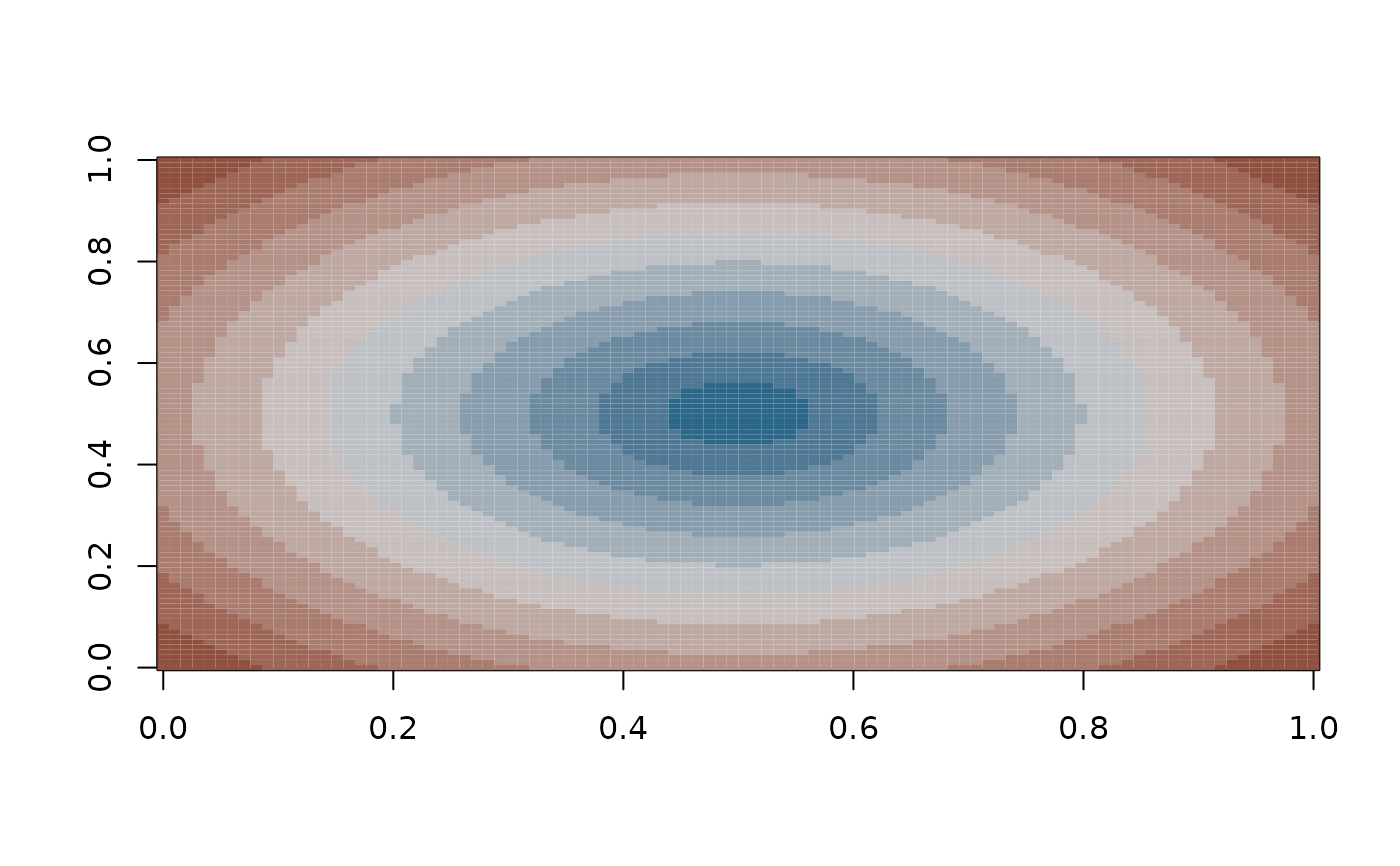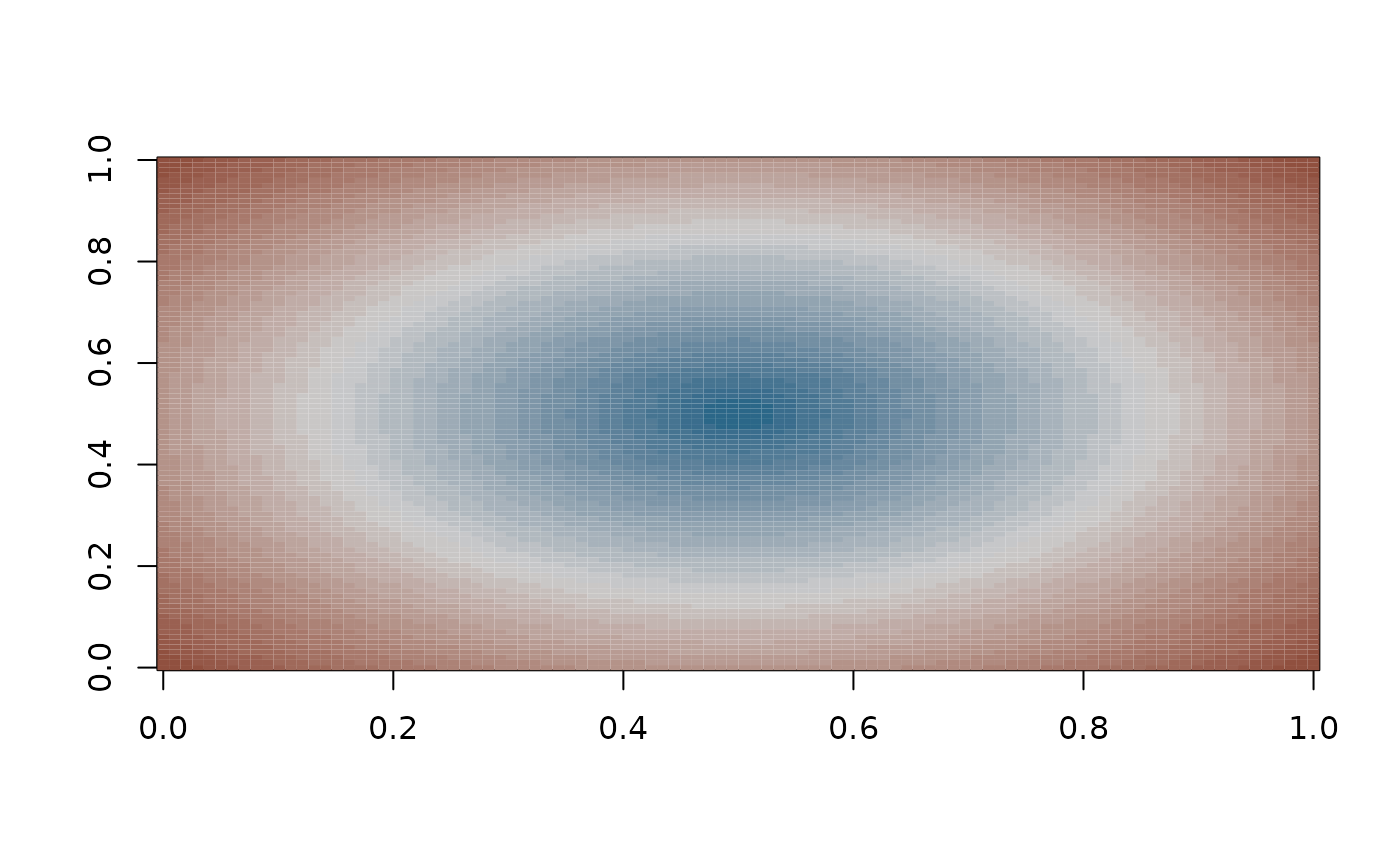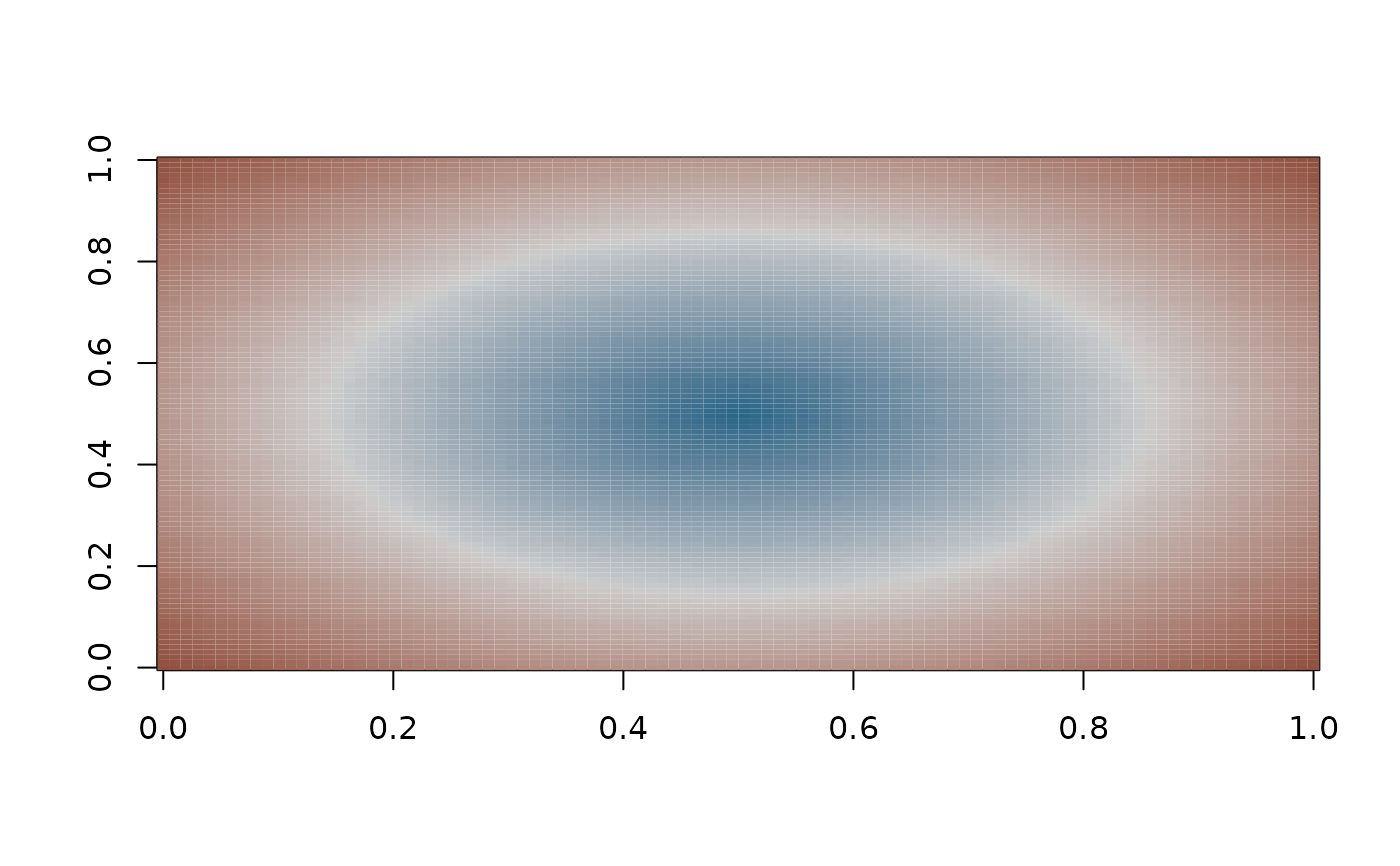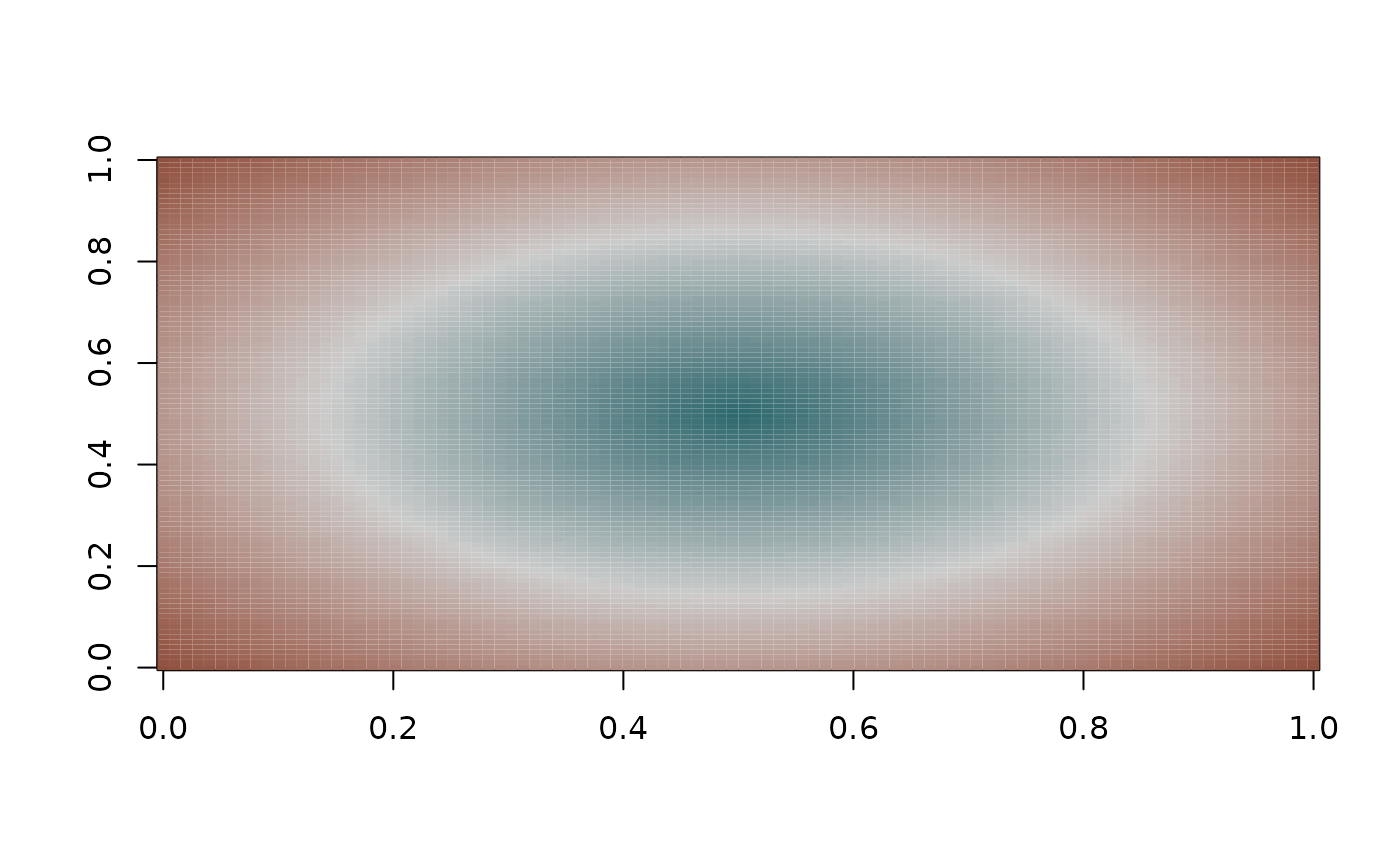Diverging colour gradient (continuous).

div_gradient_pal(
low = mnsl("10B 4/6"),
mid = mnsl("N 8/0"),
high = mnsl("10R 4/6"),
space = "Lab"
)

## Arguments

low colour for low end of gradient. colour for mid point colour for high end of gradient. colour space in which to calculate gradient. Must be "Lab" - other values are deprecated.

## Examples

x <- seq(-1, 1, length.out = 100)
r <- sqrt(outer(x^2, x^2, "+"))
image(r, col = div_gradient_pal()(seq(0, 1, length.out = 12)))image(r, col = div_gradient_pal()(seq(0, 1, length.out = 30)))image(r, col = div_gradient_pal()(seq(0, 1, length.out = 100)))library(munsell)
image(r, col = div_gradient_pal(low =
mnsl(complement("10R 4/6"), fix = TRUE))(seq(0, 1, length = 100)))